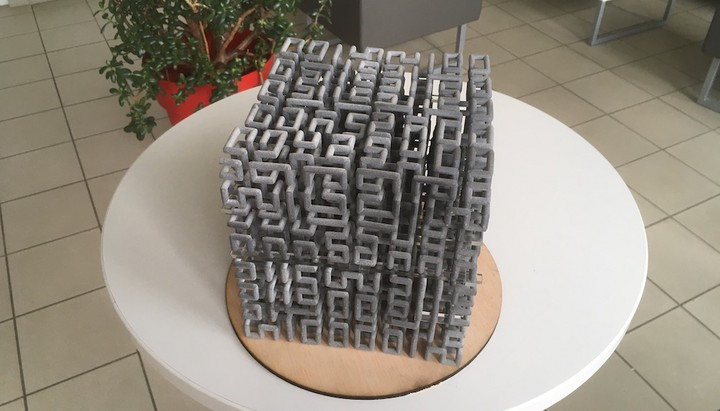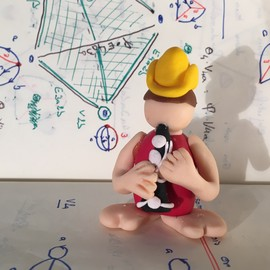# Space filling curveA three-dimensional filling curve. Design: R. Coulon

A space filling curve is a continuous map $\gamma \colon [0,1] \to \mathbb R^n$ whose range reaches every point in a higher dimensional region. Examples are the Peano curve or the Hilbert curve whose image is a unit square.

The above photo displays the four-step approximation of a three-dimensional space filling curve whose image is cube.Previous